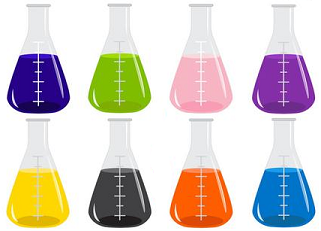### C - Cocktails

##### Languages: C, C++, Java, Python, ... (details)

Fito likes creating colorful cocktails by pouring multiple colored liquids of different densities, in such a way that the liquids don't mix. Since his friends know this, they frequently ask him to make some custom crazy colorful cocktails for them. Usually, Fito takes a lot of time figuring out if he can make such Cocktails or not. Therefore, he asked you to write a program to help him decide this automatically.He has already prepared several colored liquids with various colors and densities (two liquids may have the same color but different density). Any colorful drink consist of several color-layers. For each layer Fito uses one of the liquids he has already prepared. Morover, any cocktail he serves must satisfy the following condition:

• On top of a liquid there cannot be a liquid with higher or equal density: a layer of a colored liquid with density $x$ can be directly above the layer of a colored liquid with density $y$ only if $x$ < $y$ holds.

Fito cannot use a mixed colored liquid as a layer. For example, a new liquid with different color or density cannot be created by mixing two or more different colored liquids.For a given request, which consist of the colors each layer should have, your task is to create a program to determine whether Fito can create a cocktail with the prepared colored liquids or not.

#### Input

The input consists of a single test case. The first line consists of an integer $N$ ($1 \leq N \leq 10^5)$, which represents the number of the prepared colored liquids. The following $N$ lines consist of $C_i$ and $D_i$. $C_i$ is a string ($|C_i| <= 20$) consisting of lowercase English alphabet letters and denotes the color of the $i$-th prepared colored liquid. $D_i$ ($1 \leq D_i \leq 10^5$) is an integer and represents the density of the $i$-th prepared colored liquid.

The $N+2$ line consists of an integer $M$ ( $1 \leq M \leq 10^5$), which represents the number of color layers of the cocktail request. The following $M$ lines consists of $O_i$ ($1 \leq i \leq M$). $O_i$ is a string  ($|O_i| <= 20$)  consisting of lowercase English alphabet letters and denotes the color of the $i$-th layer from top to bottom.

#### Output

If the requested colorful drink can be served by using some of the prepared colored liquids, print 'Yes'. Otherwise, print 'No' without the quotes.

#### Sample test(s)

Input
2 blue 20 red 10 2 red blue
Output
Yes
Input
2 red 10 blue 10 2 blue red
Output
No
Input
2 red 20 blue 10 2 red orange
Output
No
Input
3 white 10 red 20 white 30 3 white red white
Output
Yes